# Real Numbers

Go back to  'Numbers'

 1 Introduction to Real Numbers 2 What are Real Numbers? 3 Properties of Real Numbers 4 Real Numbers Chart 5 Tips and Tricks on Real Numbers 6 Real Numbers Line 7 Thinking out of the Box! 8 Real Numbers for Class 10 9 Solved Examples on Real Numbers 10 Practice Questions on Real Numbers 11 Maths Olympiad Sample Papers 12 Frequently Asked Questions (FAQs) 13 FREE Worksheets of Real Numbers

We at Cuemath believe that Math is a life skill. Our Math Experts focus on the “Why” behind the “What.” Students can explore from a huge range of interactive worksheets, visuals, simulations, practice tests, and more to understand a concept in depth.

Book a FREE trial class today! and experience Cuemath’s LIVE Online Class with your child.

## Introduction

Any number that can be found in the real world is, literally, a real number.

• Counting objects gives the set of natural numbers,
$N=\{1, 2, 3, ...\}$
• The set of natural numbers along with $$0$$ would give the set of whole numbers,
$W=\{0, 1, 2, 3, ...\}$
• Dealing with debts, temperatures etc give the set of integers,
$Z = \{..., -3, -2, -1, 0, 1, 2, 3, ...\}$
•  If we cut a cake into equal pieces, then we may have a piece that represents a fraction that is an element of the set of rational numbers, $$Q$$
• The numbers that are the square roots of positive rational numbers, cube roots of rational numbers etc, say $$\sqrt{2}$$, give the set of irrational numbers, $$I$$

Among these sets, the sets $$N, W$$ and $$Z$$ are the subsets of $$Q$$.

## What are Real Numbers?

Any number, except the complex numbers, we can think of is a real number.

### Real Numbers Definition

• The set of Real Numbers is the union of the set of Rationals $$Q$$ and the set of Irrationals $$I$$
• The set of real numbers is denoted by $$R$$.
 $$R = Q \cup I$$

It means real numbers include natural numbers, whole numbers, integers, rational numbers, and irrational numbers.

Then what numbers are NOT real Numbers?

The numbers that are neither rational nor irrational, say $$\sqrt{-1}$$, are NOT real numbers. These numbers would give the set of complex numbers, $$C$$.

We can understand the same thing better from the following table:

Number set Is it a part of the set of real numbers?

Natural Numbers, $$N$$

Whole Numbers, $$W$$

Integers, $$Z$$

Rational Numbers, $$Q$$

Irrational Numbers, $$I$$

Complex Numbers, $$C$$

### Real Numbers Examples

By the above definition of the real numbers, some examples of real numbers can be $$3, 0, 1.5, \dfrac{3}{2}, \sqrt{5}, \sqrt{-9}$$,  etc.

### Are there Real Numbers that are not Rational or Irrational?

From the definition of real numbers, the set of real numbers is formed by both rational numbers and irrational numbers.

Thus there does NOT exist any real number that is neither rational nor irrational.

It simply means that if we pick up any number from $$R$$, it is either rational or irrational.

## Real Numbers Chart

We can see that the above explanation can be summarised using the following real numbers chart where some examples are also included.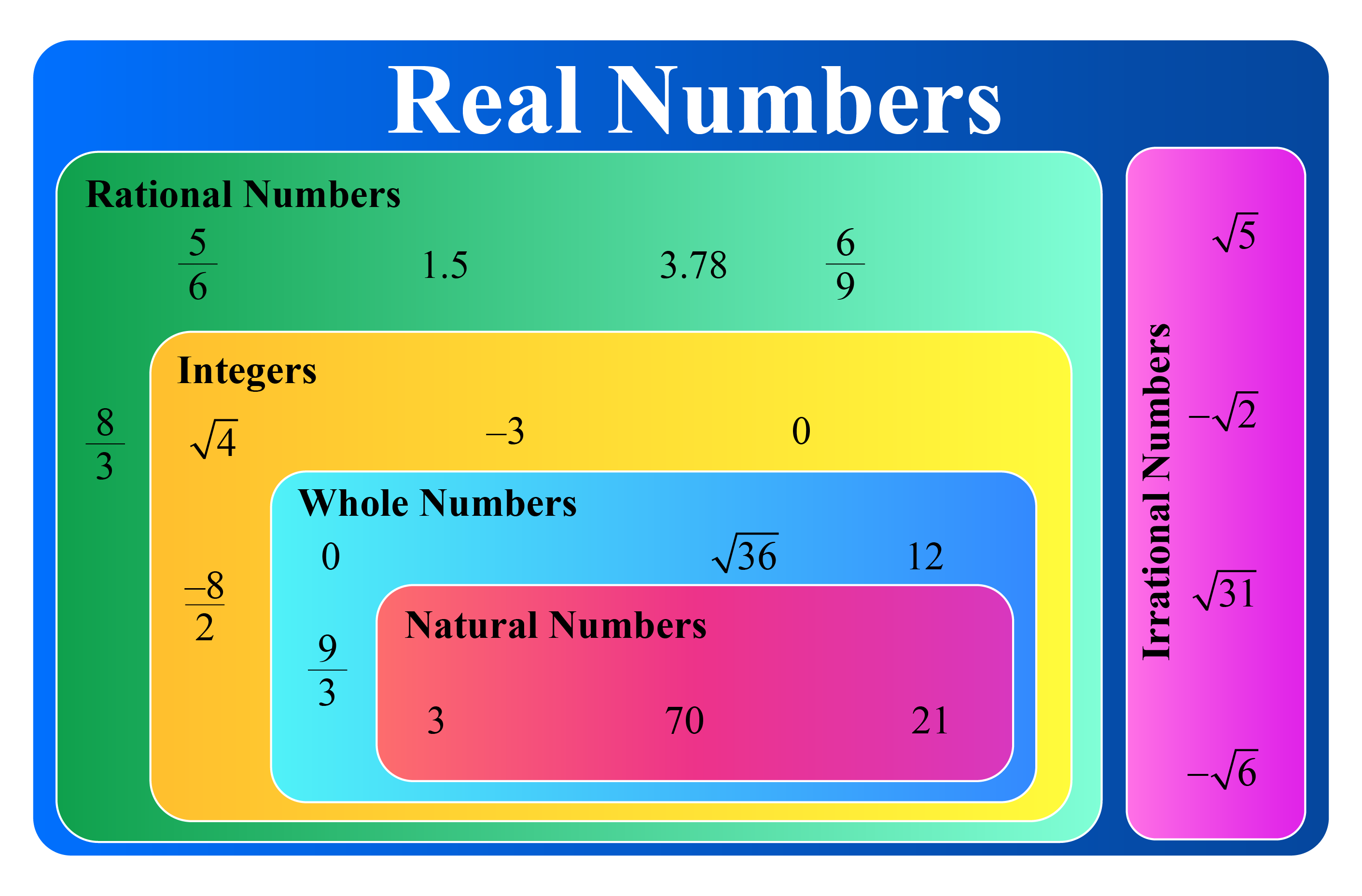We can use the following simulation to see how the set of real numbers is formed.

Click on the start button to begin the simulation.Tips and Tricks
1. All numbers except complex numbers are real numbers.
A complex number has $$i$$ in it.
For example, $$2+3i, -i$$ etc are complex numbers.

## Real Numbers Line

The number line that consists of all the Real Numbers is known as the Real Line.

The Real Line is continuous (there are no breaks or gaps or holes).

Many advanced mathematical concepts are based on the continuity of the Real Line.

We can do the Zooming Thought Experiment for the continuity of the Real Line.

• We take any two numbers on the Real Line, say $$x$$ and $$y$$, which are very close to each other.
• Each of these numbers could be either rational or irrational.
• If we were to zoom our mind’s camera onto this small, tiny interval, we would be able to find infinitely many rational numbers and infinitely many irrational numbers between these two numbers.
• Most importantly, there would be no gaps in this interval.
• It would be one nice, continuous stretch of numbers.

Thus the real number line stretches from -$$\infty$$ to $$\infty$$.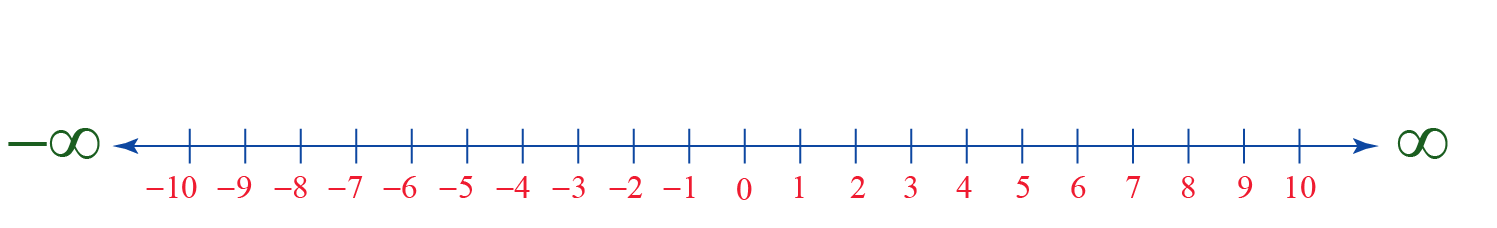### Where do Real Numbers Start From?

From the real number line, we can easily conclude that we cannot determine the number where the set of real numbers starts from.

## Properties of Real Numbers

Let’s consider any three real numbers $$a, b$$ and $$c$$.

Let’s learn the properties of real numbers using the following table:

Property Explanation Example Conclusion
Closure Property $$a+b$$ is real

$$3+2.5$$ is real

$$R$$ is closed under addition.
$$a \times b$$ is real

$$3 \times 2.5$$ is real

$$R$$ is closed under multiplication.
Associative Property

$$a+(b+c) = (a+b)+c$$

$$2.5 + (1+ 6) = (2.5+1)+6$$ $$R$$ is associative under addition.
$$a \times (b \times c) = (a\times b)\times c$$ $$2.5 \times(1 \times 6) = (2.5 \times 1) \times 6$$

$$R$$ is associative under multiplication.

Commutative Property

$$a+b$$ = $$b+a$$

$$3+2.5$$ = $$2.5 + 3$$

$$R$$ is commutative under addition.

$$a \times b$$ = $$b \times a$$

$$3 \times 2.5= 2.5 \times 3$$ $$R$$ is commutative under multiplication.

$$a+0=0+a=a$$

Here $$0$$ is the additive identity.

$$2+0=0+2=2$$ Additive identity exists in $$R$$.

Existence of Multiplicative Identity

$$a \times 1 = 1 \times a = a$$

Here $$1$$ is the multiplicative identity.

$$2 \times 1 = 1 \times 2 =2$$ Multiplicative identity exists in $$R$$.

$$a+(-a)= (-a)+a=0$$

Here, $$a$$ and $$-a$$ are the additive inverses of each other.

$$2+(-2) = (-2)+2=0$$

Here, $$2$$ and $$-2$$ are additive inverses of each other.

Every element of $$R$$ has its additive inverse in $$R$$.

Existence of Multiplicative Inverse

$$a \times \frac{1}{a} = \frac{1}{a} \times a=1$$

Here, $$a$$ and $$\frac{1}{a}$$ are the multiplicative inverses of each other.

$$2 \times \frac{1}{2} = \frac{1}{2} \times 2=1$$

Here, $$2$$ and $$\frac{1}{2}$$ are the multiplicative inverses of each other.

Every element of $$R$$, except $$0$$, has its multiplicative inverse in $$R$$.Think Tank
1. Is $$- \dfrac{3}{2}$$ a real number?
2. Which is a real number? $$\sqrt{4}$$  or $$\sqrt{-5}$$
3. Is $$R$$ closed under subtraction and division?
4. Is $$R$$ associative under subtraction and division?
5. Is $$R$$ commutative under subtraction and division?

## Real Numbers for Class 10:

The chapter “Real Numbers” of Class 10 mainly deals with two things:

### Euclid’s Division Lemma

The Euclid’s division lemma says the following:

“For any two positive integers $$a$$ and $$b$$ there exist two whole numbers $$q$$ and $$r$$ such that $$a= bq+r$$, where $$0 \leq r<b$$”.

Got confused with this? We can understand this using the following example:

Let’s divide $$37$$ by $$5$$. This means that $$a=37$$ and $$b=5$$. We see what we get: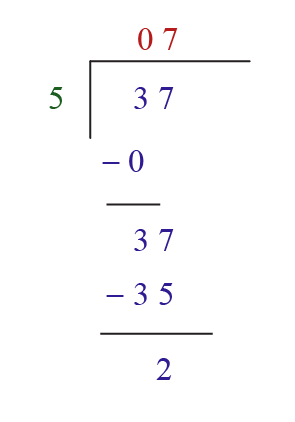Here, the divisor is, $$b=5$$

The dividend is, $$a=37$$

The quotient is, $$q=7$$

The remainder is, $$r= 2$$

By using the checking method of division which we learnt in our previous classes:

 $\text{Dividend} = \text{Divisor} \times \text{Quotient} + \text{Remainder}$

Substituting all the values here, we get:

$a = bq + r$

i.e;

$37 = 5 \times 7 + 2$

Let’s understand this lemma using the following simulation.

Enter the values for $$a$$ and $$b$$ and click Go to start the simulation:

Isn’t Euclid’s division lemma easy for you now?

### The Fundamental Theorem of Arithmetic

The Fundamental Theorem of Arithmetic says the following:

“Any composite number can be represented as the product of prime numbers where this product is unique, neglecting the order of the factors”.

Example:

Express $$240$$ as the product of prime factors.

Solution:

We will find the prime factorisation of $$240$$ as follows: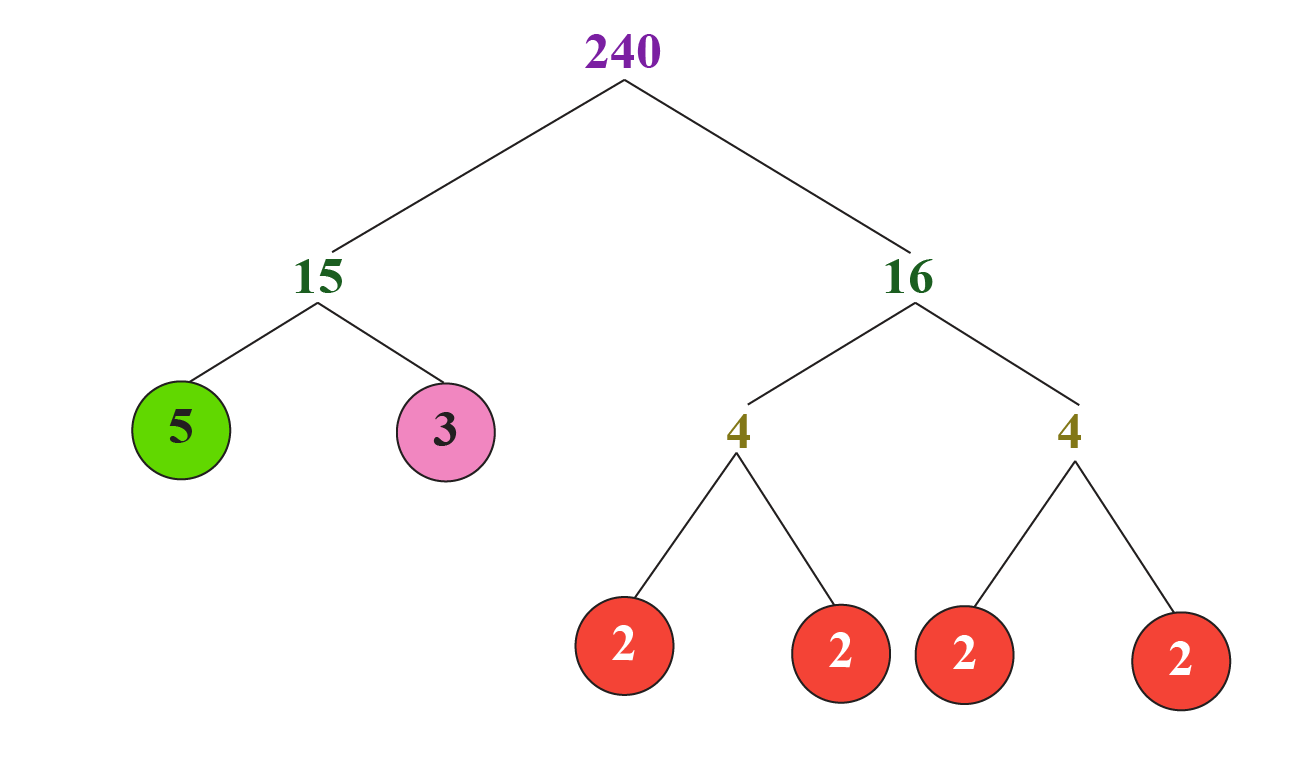$$240 = 2^{4} \times 3^{1} \times 5^{1}$$

You can go ahead and enter a number here to see how to find its prime factorisation.

Click the Go button once you enter your number:

Help your child score higher with Cuemath’s proprietary FREE Diagnostic Test. Get access to detailed reports, customised learning plans and a FREE counselling session. Attempt the test now.

## Solved Examples

 Example 1

Identify the real numbers among the following numbers.
$\sqrt{6}, -3, 3.15, \frac{-1}{2}, \sqrt{-5}$

Solution:

Among the given numbers, $$\sqrt{-5}$$ is a complex number. So it cannot be a real number.

The other numbers are either rational or irrational and hence they are real numbers.

 $$\text{Real numbers are } \sqrt{6}, -3, 3.15 \text{ and } \frac{-1}{2}$$
 Example 2

Use Euclid’s division algorithm to find the HCF of $$135$$ and $$225$$.

Solution:

You have to find the HCF of given integers by using Euclid’s Division Lemma.

It is a technique to compute the highest common factor of two given positive integers.

Recall that the HCF of two positive integers $$a$$ and $$b$$ is the largest positive integer that divides both $$a$$ and $$b$$.

To obtain the HCF of two positive integers say $$a$$ and $$b$$ with $$a>b$$ follow the below steps:

Step 1: Apply Euclid’s division lemma to $$a$$ and $$b$$. So we find whole numbers $$q$$ and $$r$$ such that

$a=b q+r, 0 \leq r<b$

Step 2: If $$r=0$$, then $$b$$ is the HCF of $$a$$ and $$b$$. If $$r \neq 0$$, apply the division lemma to $$b$$ and $$r$$.

Step 3: Continue the process till the remainder is zero. The divisor at this stage will be the required HCF.

In this case, $$225>135$$. We apply Euclid’s division lemma to $$135$$ and $$225$$ and get:

$225=(135 \times 1)+90$

Since, the remainder $$r \neq 0$$, we apply the division lemma to $$135$$ and $$90$$ to get:

$135=(90 \times 1)+45$

Now, we consider $$90$$ as the divisor and $$45$$ as the remainder and apply the division lemma, to get:

$90=(45 \times 2)+0$

Since the remainder is zero and the divisor is $$45$$, therefore, the HCF of $$135$$ and $$225$$ is $$45$$.

 $$HCF$$ $$(135, 225)= 45$$
 Example 3

Express $$156$$ as the product of primes.

Solution:

We first prime factorise the given number $$156$$ using the factor tree.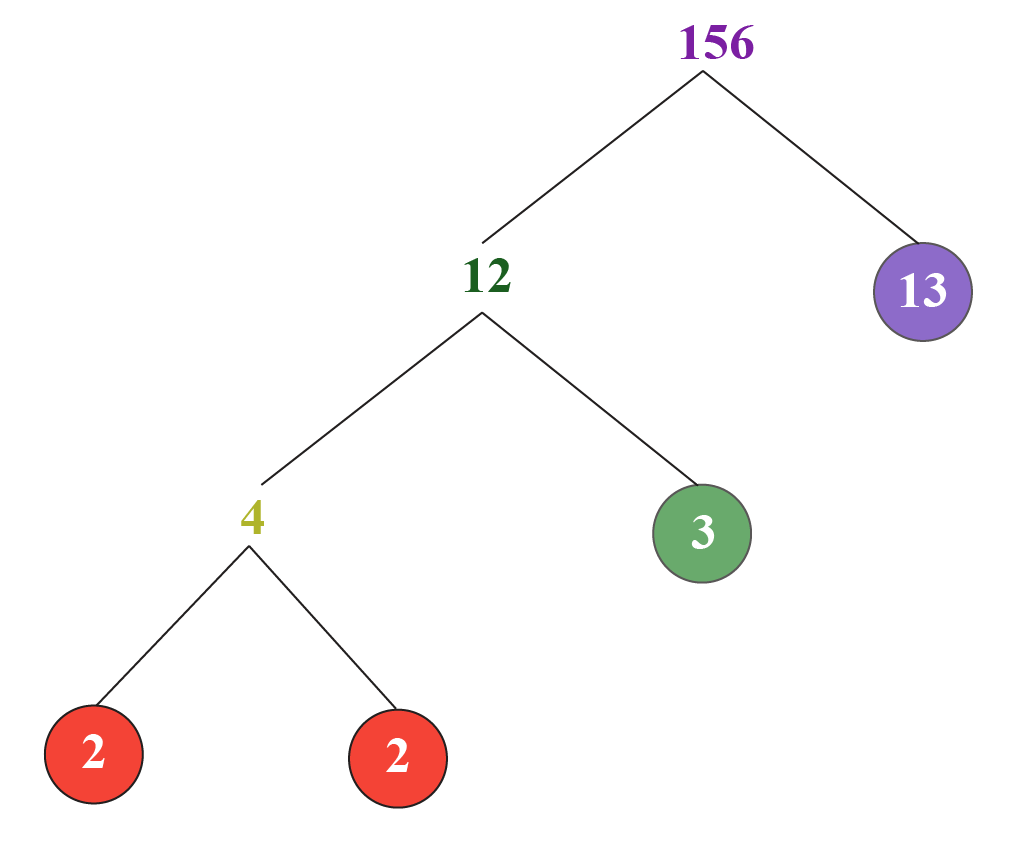Thus,
$156 = 2 \times 2 \times 3 \times 13$

 $$156= 2^2 \times 3^1 \times 13^1$$
CLUEless in Math? Check out how CUEMATH Teachers will explain Real Numbers to your kid using interactive simulations & worksheets so they never have to memorise anything in Math again!

Explore Cuemath Live, Interactive & Personalised Online Classes to make your kid a Math Expert. Book a FREE trial class today!

## Practice Questions

Here are a few activities for you to practice. Select/Type your answer and click the "Check Answer" button to see the result.

IMO (International Maths Olympiad) is a competitive exam in Mathematics conducted annually for school students. It encourages children to develop their math solving skills from a competition perspective.

## 1. What are real numbers with examples?

The set of Real Numbers is the union of the set of Rationals $$Q$$ and Irrationals $$I$$.

Some real numbers examples can be $$-2, 3.15, 0, \frac{3}{2}, \sqrt{21}, \sqrt{-3}$$, etc.

## 2. What is a real number?

Any number other than a complex number is a real number. The set of Real Numbers is defined as the union of the set of Rationals Q and the set of Irrationals I.

The set of Real Numbers is denoted by the letter $$R$$.

## 3. Is $$0$$ a real number?

$$0$$ is a real number because it belongs to the set of whole numbers, and the set of whole numbers is a subset of real numbers.

## 4. What is not a real number?

Complex numbers, say $$\sqrt{-1}$$, are not real numbers. In other words, the numbers that are neither rational nor irrational, say \sqrt{-1}, are NOT real numbers. These numbers would give us the set of complex numbers, $$C$$.

Numbers and Number Systems
grade 9 | Questions Set 2
Numbers and Number Systems
Numbers and Number Systems
grade 9 | Questions Set 1
Numbers and Number Systems